## Steinmetz Solid

The solid common to two (or three) right circular Cylinders of equal Radii intersecting at Right Angles is called the Steinmetz solid. (Two Cylinders intersecting at Right Angles are sometimes called a Bicylinder, and three intersecting Cylinders a Tricylinder.)

The Volume common to two intersecting right Cylinders of Radiusis(1)

If the two right Cylinders are of different Radiiandwith, then the Volume common to them is(2)

whereis the complete Elliptic Integral of the First Kind,is the complete Elliptic Integral of the Second Kind, andis the Modulus.

The curves of intersection of two cylinders of Radiiand, shown above, are given by the parametric equations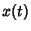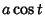(3)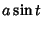(4)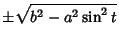(5)

(Gray 1993).

The Volume common to two Elliptic Cylinders(6)

withis(7)

where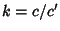(Bowman 1961, p. 34).

For three Cylinders of Radiiintersecting at Right Angles, the Volume of intersection is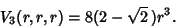(8)

References

Bowman, F. Introduction to Elliptic Functions, with Applications. New York: Dover, 1961.

Gardner, M. The Unexpected Hanging and Other Mathematical Diversions. Chicago, IL: Chicago University Press, pp. 183-185, 1991.

Gray, A. Modern Differential Geometry of Curves and Surfaces. Boca Raton, FL: CRC Press, pp. 149-150, 1993.

Wells, D. G. #555 in The Penguin Book of Curious and Interesting Puzzles. London: Penguin Books, 1992.